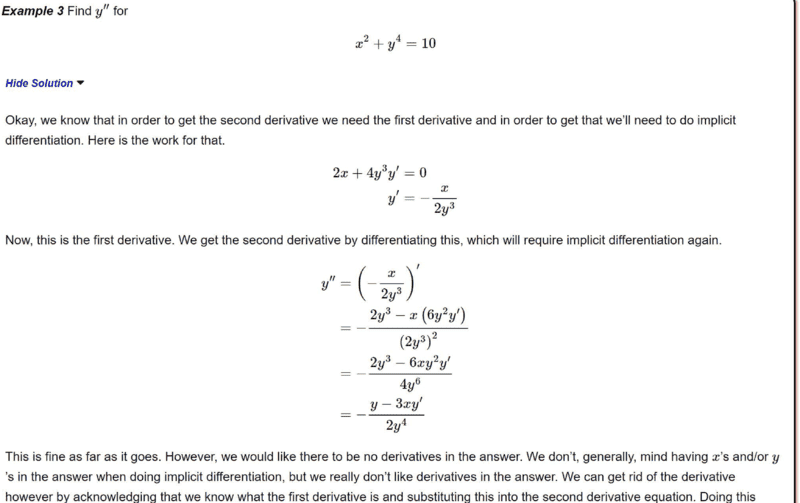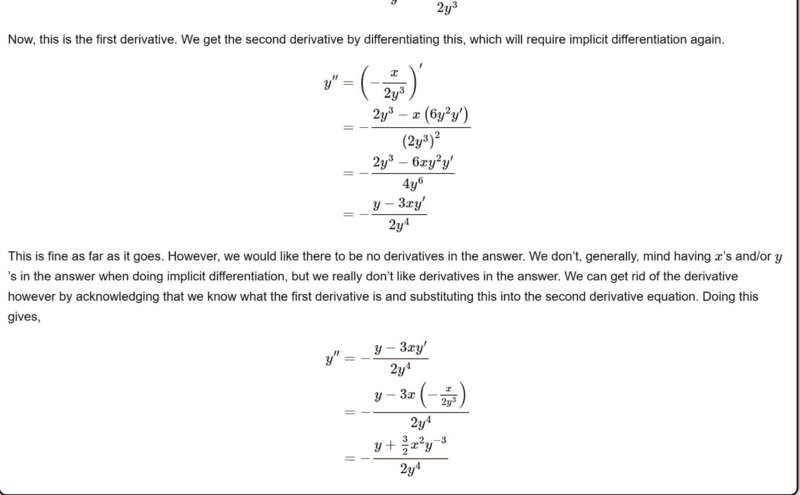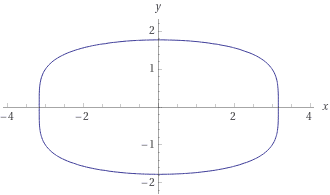# Find the second derivative of the relation; ##x^2+y^4=10##

• chwala

#### chwala

Gold Member
Homework Statement
Find the second derivative, ##\dfrac{d^2y}{dx^2}## for the relation;

##x^2+y^4=10##
Relevant Equations
differentiation
Find text (question and working to solution here ...this is very clear to me...on the use of implicit differentiation and quotient rule to solution). I am seeking an alternative approach.Now from my study we can also have; using partial derivatives...

##\dfrac{d^2y}{dx^2}=\dfrac{-1}{2y^3}+\left[\dfrac{-x}{2}⋅\dfrac{-3}{y^4}⋅\dfrac{-x}{2y^3}\right]##

##\dfrac{d^2y}{dx^2}=-\dfrac{1}{2y^3}-\dfrac{3x^2}{4y^7}##

##\dfrac{d^2y}{dx^2}=\dfrac{-2y^4-3x^2}{2y^7}##

##\dfrac{d^2y}{dx^2}=-\left[\dfrac{2y^4+3x^2}{2y^7}\right]##

your thoughts...any other approach is welcome guys...

the text book solution is;

##\dfrac{d^2y}{dx^2}=-\left[\dfrac{y+1.5x^2y^{-3}}{2y^4}\right]## ...just in case it is not visible enough...

Last edited:
•Delta2
Ehm, can't understand how you got your first equation using partial derivatives. Can you show the in between steps?

Partial derivatives

is that a partial derivative?

wha do you want to do? Not use implicit differentiation? Then solve y as function of x and use chain rule etc.

••bob012345 and Delta2
Differentiatng $$2x+4y^3y'=0$$ by x again,
$$2+12y^2y'^2+4y^3y^"=0$$
$$y^"=-\frac{2+12y^2y'^2}{4y^3}=-\frac{2+3x^2y^{-4}}{4y^3}=-\frac{2+3(10-y^4)y^{-4}}{4y^3}=\frac{1-30y^{-4}}{4y^3}$$
as a function of y.

Last edited:
•chwala and Delta2
Ehm, can't understand how you got your first equation using partial derivatives. Can you show the in between steps?
##\dfrac{dy}{dx}=-\dfrac{x}{2y^3}## Let ##\dfrac{dy}{dx}=m(x,y)##

##\dfrac{d^2y}{dx^2}=\dfrac{∂g}{∂x}+ \dfrac{∂g}{∂y}m(x,y)##

•Delta2
Differentiatng $$2x+4y^3y'=0$$ by x again,
$$2+12yy'^2+4y^3y^"=0$$
$$y^"=-\frac{2+12y^2y'^2}{4y^3}=-\frac{2+3x^2y^{-4}}{4y^3}=-\frac{2+3(10-y^4)^2y^{-4}}{4y^3}$$

Aaaaaaaargh i have been picketing on this approach for some time...i could not see

##2+12yy'^2+4y^3y^"=0## ... i was getting ##y^{'}## at that point instead of ##(y^{'})^2## lol...this implies as i had earlier thought to myself that one can use implicit differentiation and product rule to the required solution!

i have been navigating around this approach for a long time...... i was getting;

##2+12y^2y^{'}+4y^3y^{''}=0##... !

thanks @anuttarasammyak##2+12y^2y^{'}y^{'}+4y^3y^{''}=0##

##=2+12y^2y^{'2}+4y^3y^{''}=0##

cheers man!

I can see you just amended this;

##2+12yy'^2+4y^3y^"=0## to

##2+12y^2y'^2+4y^3y^"=0##

just to clarify to those who may be following the thread (thus avoid confusion) as i had earlier quoted your post...cheers @anuttarasammyak

Last edited:
##\dfrac{dy}{dx}=-\dfrac{x}{2y^3}## Let ##\dfrac{dy}{dx}=m(x,y)##

##\dfrac{d^2y}{dx^2}=\dfrac{∂g}{∂x}+ \dfrac{∂g}{∂y}m(x,y)##
you need to fix it a bit, what is function g?

you need to fix it a bit, what is function g?
A minute...

you need to fix it a bit, what is function g?
##\dfrac{d^2y}{dx^2}=\dfrac{∂m}{∂x}+ \dfrac{∂m}{∂y}m(x,y)##

•Delta2
Thread title: "Find the second derivative of the function ##x^2+y^4=10##"

That's a relation, not a function. If we solve for y in terms of x, we get $$y = \pm \sqrt{10 - x^2}$$, which represents two functions.

If you want a different approach differentiate both sides of this equation to get dy/dx, and then differentiate once again.

•SammyS, chwala, Delta2 and 1 other person
Thread title: "Find the second derivative of the function ##x^2+y^4=10##"

That's a relation, not a function. If we solve for y in terms of x, we get $$y = \pm \sqrt{10 - x^2}$$, which represents two functions.

If you want a different approach differentiate both sides of this equation to get dy/dx, and then differentiate once again.
Let me fix that...true.

Now from my study ...

##\dfrac{d^2y}{dx^2}=-\left[\dfrac{2y^4+3x^2}{2y^7}\right]##

your thoughts...any other approach is welcome guys...

the text book solution is;

##\dfrac{d^2y}{dx^2}=-\left[\dfrac{y+1.5x^2y^{-3}}{2y^4}\right]## ...just in case it is not visible enough...
Your result is equivalent to the following:
##\dfrac{d^2y}{dx^2}=-\left[ \dfrac{2y^4}{2y^7} + \dfrac{3x^2}{2y^7} \right]
=-\left[ \dfrac{1}{y^3} + \dfrac{3x^2}{2y^7} \right]\ ,##

whereas the textbook solution is equivalent to:
##\dfrac{d^2y}{dx^2}=-\left[ \dfrac{y}{2y^4}+\dfrac{3x^2y^{-3}}{4y^4} \right]=\dfrac{-1}{\ 2}\left[ \dfrac{1}{y^3} + \dfrac{3x^2}{2y^7} \right]## .

•chwala and Delta2
Let me fix that...true.
@Mark44 In reference to your post ##10##; This will be a different second- order derivative from our envisaged one...because of the constant ##10##. The derivative will be explicitly in terms of ##x## and the working to solution would make use of chain rule.

•Delta2
Your result is equivalent to the following:
##\dfrac{d^2y}{dx^2}=-\left[ \dfrac{2y^4}{2y^7} + \dfrac{3x^2}{2y^7} \right]
=-\left[ \dfrac{1}{y^3} + \dfrac{3x^2}{2y^7} \right]\ ,##

whereas the textbook solution is equivalent to:
##\dfrac{d^2y}{dx^2}=-\left[ \dfrac{y}{2y^4}+\dfrac{3x^2y^{-3}}{4y^4} \right]=\dfrac{-1}{\ 2}\left[ \dfrac{1}{y^3} + \dfrac{3x^2}{2y^7} \right]## .
Interesting, why does it misses a factor of 1/2, I don't see anything wrong with his approach?

Interesting, why does it misses a factor of 1/2, I don't see anything wrong with his approach?
Something wrong somewhere, I may need to check @SammyS Working...I checked both solutions with arbitrary values of ##x## and ##y## and they were both correct. The two derivatives were giving same values...which means there could be something wrong here...

##x^2+y^4=10##Last edited:
•chwala and SammyS
Interesting, why does it misses a factor of 1/2, I don't see anything wrong with his approach?
OP (@chwala ) has an error in going from his second line to his third line.

##\dfrac{d^2y}{dx^2}=-\dfrac{1}{2y^3}-\dfrac{3x^2}{4y^7}##

##\dfrac{d^2y}{dx^2}=\dfrac{-2y^4-3x^2}{2y^7}##

••chwala and Delta2
OP (@chwala ) has an error in going from his second line to his third line.

##\dfrac{d^2y}{dx^2}=-\dfrac{1}{2y^3}-\dfrac{3x^2}{4y^7}##

##\dfrac{d^2y}{dx^2}=\dfrac{-2y^4-3x^2}{2y^7}##
Yes I just saw it...let me repost ...

Thx @SammyS so it was an ordinary algebraic error and I was seeking for some weird calculus error with the partial derivative chain rule...

Homework Statement:: Find the second derivative, ##\dfrac{d^2y}{dx^2}## for the relation;

##x^2+y^4=10##
Relevant Equations:: differentiation

Find text (question and working to solution here ...this is very clear to me...on the use of implicit differentiation and quotient rule to solution). I am seeking an alternative approach.

View attachment 303783

View attachment 303784

Now from my study we can also have; using partial derivatives...

##\dfrac{d^2y}{dx^2}=\dfrac{-1}{2y^3}+\left[\dfrac{-x}{2}⋅\dfrac{-3}{y^4}⋅\dfrac{-x}{2y^3}\right]##

##\dfrac{d^2y}{dx^2}=-\dfrac{1}{2y^3}-\dfrac{3x^2}{4y^7}##

##\dfrac{d^2y}{dx^2}=\dfrac{-2y^4-3x^2}{2y^7}##

##\dfrac{d^2y}{dx^2}=-\left[\dfrac{2y^4+3x^2}{2y^7}\right]##

your thoughts...any other approach is welcome guys...

the text book solution is;

##\dfrac{d^2y}{dx^2}=-\left[\dfrac{y+1.5x^2y^{-3}}{2y^4}\right]## ...just in case it is not visible enough...
Error on denominator ought to be ##4y^7## and not ##2y^7##... Will amend later once I get on my computer...

Correct working is as follows...

##\dfrac{d^2y}{dx^2}=\dfrac{-1}{2y^3}+\left[\dfrac{-x}{2}⋅\dfrac{-3}{y^4}⋅\dfrac{-x}{2y^3}\right]##

##\dfrac{d^2y}{dx^2}=-\dfrac{1}{2y^3}-\dfrac{3x^2}{4y^7}##

##\dfrac{d^2y}{dx^2}=\dfrac{-2y^4-3x^2}{4y^7}##

##\dfrac{d^2y}{dx^2}=-\left[\dfrac{2y^4+3x^2}{4y^7}\right]##

At times i work on math problems when i am really tired but no excuse for such an error. Cheers guys...Last edited:
@Mark44 In reference to your post ##10##; This will be a different second- order derivative from our envisaged one...because of the constant ##10##. The derivative will be explicitly in terms of ##x## and the working to solution would make use of chain rule.
I guess what you mean is that the second derivative shown in the textbook answer involves both x and y, while the technique I described gives both the derivative and second derivative as functions of x alone.

These two answers can be reconciled by algebraic means, by solving for y in the equation ##x^2 + y^4 = 10##, and substituting into the textbook's solution. This same technique is often useful in verifying that work done in implicit differentiation is correct. IOW, after one has found a derivative using implicit differentiation, go back to the original equation, solve for the dependent variable (if possible), and take the derivative by normal means.

•chwala
I guess what you mean is that the second derivative shown in the textbook answer involves both x and y, while the technique I described gives both the derivative and second derivative as functions of x alone.

These two answers can be reconciled by algebraic means, by solving for y in the equation ##x^2 + y^4 = 10##, and substituting into the textbook's solution. This same technique is often useful in verifying that work done in implicit differentiation is correct. IOW, after one has found a derivative using implicit differentiation, go back to the original equation, solve for the dependent variable (if possible), and take the derivative by normal means.
Ok, i hear you...you are talking of something else though...solving for ##y## or finding second -derivative? for purposes of verification...

I guess your assumption here is that the solution i.e the second-derivative done implicitly has already been given. Supposing you do not have the solution ( that takes me back to my earlier question) then its clear that this approach may not be useful. Its only useful if you have the solution and you want to do some verification as you clearly put it. Cheers mate.

Ok, i hear you...you are talking of something else though...solving for y or finding second -derivative? for purposes of verification...
I'm talking about another form of differentiation. The solution in the problem you posted uses implicit differentiation. Both of the derivatives they calculated were given as functions of both x and y. "Implicit" here means that although you're dealing with a relation (not a function), the assumption is that, in some suitably small neighborhood, you can consider y to be some function of x alone, but not stated -- only implied.

The problem as given is a natural for implicit differentiation. What I described was different, relying on ordinary single-variable differentiation. The difference is that you need to start off with the function formula that involves only one variable. I showed that in post #10. After that, just use the rules of differentiation to get the two derivatives. Since the problem didn't specify what method to use, my approach would have been permitted.
I guess your assumption here is that the solution i.e the second-derivative done implicitly has already been given. Supposing you do not have the solution ( that takes me back to my earlier question) then its clear that this approach may not be useful. Its only useful if you have the solution and you want to do some verification as you clearly put it. Cheers mate.
What I meant was, here is a second approach, one that could be used to solve the problem, or verify that a solution done in a different way is correct. In most areas of mathematics where you calculate something, it's always useful and sometimes necessary to check your work.

I'm talking about another form of differentiation. The solution in the problem you posted uses implicit differentiation. Both of the derivatives they calculated were given as functions of both x and y. "Implicit" here means that although you're dealing with a relation (not a function), the assumption is that, in some suitably small neighborhood, you can consider y to be some function of x alone, but not stated -- only implied.

The problem as given is a natural for implicit differentiation. What I described was different, relying on ordinary single-variable differentiation. The difference is that you need to start off with the function formula that involves only one variable. I showed that in post #10. After that, just use the rules of differentiation to get the two derivatives. Since the problem didn't specify what method to use, my approach would have been permitted.

What I meant was, here is a second approach, one that could be used to solve the problem, or verify that a solution done in a different way is correct. In most areas of mathematics where you calculate something, it's always useful and sometimes necessary to check your work.
True, I now get it. The problem didn't specify which particular method to use...and that means your approach is correct.

as a function of y.
As a function of x
$$y^"=A\frac{x^2+20}{4(10-x^2)^{7/4}}$$
where A=-1 for the y(x) curve over x-axis and 1 for the y(x) curve under x-axis referring the figure in #16.

Last edited:
•chwala